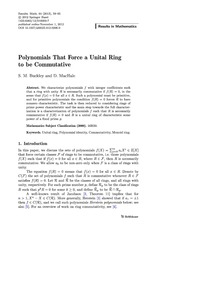# Polynomials That Force a Unital Ring to be Commutative

Buckley, Stephen M. and MacHale, Desmond (2013) Polynomials That Force a Unital Ring to be Commutative. Results in Mathematics, 64 (1-2). pp. 59-65. ISSN 1422-6383Previewmore...Add this article to your Mendeley library

## Abstract

We characterize polynomials f with integer coefficients such that a ring with unity R is necessarily commutative if f(R) = 0, in the sense that f(x) = 0 for all x∈R . Such a polynomial must be primitive, and for primitive polynomials the condition f(R) = 0 forces R to have nonzero characteristic. The task is then reduced to considering rings of prime power characteristic and the main step towards the full characterization is a characterization of polynomials f such that R is necessarily commutative if f(R) = 0 and R is a unital ring of characteristic some power of a fixed prime p.

Item Type: Article 16R50; Unital ring; Polynomial identity; Commutativity; Monoid ring; Faculty of Science and Engineering > Mathematics and Statistics 4829 https://doi.org/10.1007/s00025-012-0296-0 Prof. Stephen Buckley 18 Mar 2014 12:15 Results in Mathematics Springer Verlag (Germany) YesItem control page